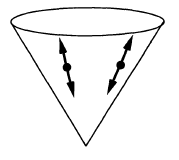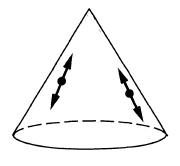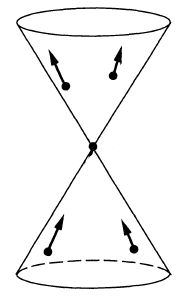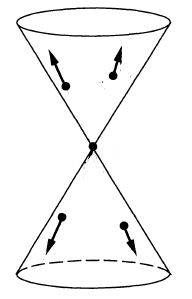# nLab Dirac propagator

Contents

### Context

#### Differential geometry

synthetic differential geometry

Introductions

from point-set topology to differentiable manifolds

Differentials

V-manifolds

smooth space

Tangency

The magic algebraic facts

Theorems

Axiomatics

cohesion

• (shape modality $\dashv$ flat modality $\dashv$ sharp modality)

$(\esh \dashv \flat \dashv \sharp )$

• dR-shape modality$\dashv$ dR-flat modality

$\esh_{dR} \dashv \flat_{dR}$

infinitesimal cohesion

tangent cohesion

differential cohesion

singular cohesion

$\array{ && id &\dashv& id \\ && \vee && \vee \\ &\stackrel{fermionic}{}& \rightrightarrows &\dashv& \rightsquigarrow & \stackrel{bosonic}{} \\ && \bot && \bot \\ &\stackrel{bosonic}{} & \rightsquigarrow &\dashv& \mathrm{R}\!\!\mathrm{h} & \stackrel{rheonomic}{} \\ && \vee && \vee \\ &\stackrel{reduced}{} & \Re &\dashv& \Im & \stackrel{infinitesimal}{} \\ && \bot && \bot \\ &\stackrel{infinitesimal}{}& \Im &\dashv& \& & \stackrel{\text{étale}}{} \\ && \vee && \vee \\ &\stackrel{cohesive}{}& \esh &\dashv& \flat & \stackrel{discrete}{} \\ && \bot && \bot \\ &\stackrel{discrete}{}& \flat &\dashv& \sharp & \stackrel{continuous}{} \\ && \vee && \vee \\ && \emptyset &\dashv& \ast }$

Models

Lie theory, ∞-Lie theory

differential equations, variational calculus

Chern-Weil theory, ∞-Chern-Weil theory

Cartan geometry (super, higher)

# Contents

## Idea

What is called the Dirac propagator is the Green functions for the wave operator/Klein-Gordon operator (hence “propagator”) on a globally hyperbolic spacetime $(X,e)$ which is the sum of the advanced propagator $\Delta_A$ and the retarded propagator $\Delta_R$

$\Delta_D \coloneqq \Delta_R + \Delta_A$

Since $\Delta_A(x,y) = \Delta_R(y,x)$, this is symmetric in its arguments, reflecting the fact that this is the integral kernel for time-ordered products away from the diagonal.

propagators (i.e. integral kernels of Green functions)
for the wave operator and Klein-Gordon operator
on a globally hyperbolic spacetime such as Minkowski spacetime:

namesymbolwave front setas vacuum exp. value
of field operators
as a product of
field operators
causal propagator\begin{aligned}\Delta_S & = \Delta_+ - \Delta_- \end{aligned}$\phantom{A}\,\,\,-$\begin{aligned} & i \hbar \, \Delta_S(x,y) = \\ & \left\langle \;\left[\mathbf{\Phi}(x),\mathbf{\Phi}(y)\right]\; \right\rangle \end{aligned}Peierls-Poisson bracket
advanced propagator$\Delta_+$\begin{aligned} & i \hbar \, \Delta_+(x,y) = \\ & \left\{ \array{ \left\langle \; \left[ \mathbf{\Phi}(x),\mathbf{\Phi}(y) \right] \; \right\rangle &\vert& x \geq y \\ 0 &\vert& y \geq x } \right. \end{aligned}future part of
Peierls-Poisson bracket
retarded propagator$\Delta_-$\begin{aligned} & i \hbar \, \Delta_-(x,y) = \\ & \left\{ \array{ \left\langle \; \left[\mathbf{\Phi}(x),\mathbf{\Phi}(y) \right] \; \right\rangle &\vert& y \geq x \\ 0 &\vert& x \geq y } \right. \end{aligned}past part of
Peierls-Poisson bracket
Wightman propagator\begin{aligned} \Delta_H &= \tfrac{i}{2}\left( \Delta_+ - \Delta_-\right) + H\\ & = \tfrac{i}{2}\Delta_S + H \\ & = \Delta_F - i \Delta_- \end{aligned}\begin{aligned} & \hbar \, \Delta_H(x,y) \\ & = \left\langle \; \mathbf{\Phi}(x) \mathbf{\Phi}(y) \; \right\rangle \\ & = \underset{ = 0 }{\underbrace{\left\langle \; : \mathbf{\Phi}(x) \mathbf{\Phi}(y) : \; \right\rangle}} \\ & \phantom{=} + \left\langle \; \left[ \mathbf{\Phi}^{(-)}(x), \mathbf{\Phi}^{(+)}(y) \right] \; \right\rangle \end{aligned}positive frequency of
Peierls-Poisson bracket,
Wick algebra-product,
2-point function
$\phantom{=}$ of vacuum state
$\phantom{=}$ or generally of
$\phantom{=}$ Hadamard state
Feynman propagator\begin{aligned}\Delta_F & = \tfrac{i}{2}\left( \Delta_+ + \Delta_- \right) + H \\ & = i \Delta_D + H \\ & = \Delta_H + i \Delta_- \end{aligned}\begin{aligned} & \hbar \, \Delta_F(x,y) \\ & = \left\langle \; T\left( \; \mathbf{\Phi}(x)\mathbf{\Phi}(y) \;\right) \; \right\rangle \\ & = \left\{ \array{ \left\langle \; \mathbf{\Phi}(x)\mathbf{\Phi}(x) \; \right\rangle &\vert& x \geq y \\ \left\langle \; \mathbf{\Phi}(y) \mathbf{\Phi}(x) \; \right\rangle &\vert& y \geq x } \right.\end{aligned}time-ordered product

What is now called the Dirac propagator was first considered in

• Paul Dirac, Classical theory of radiating electrons, Proc. Roy. Soc. A 167 (1983) 148-169

An overview of the Green functions of the Klein-Gordon operator, hence of the Feynman propagator, advanced propagator, retarded propagator, causal propagator etc. is given in

• Mikica Kocic, Invariant Commutation and Propagation Functions Invariant Commutation and Propagation Functions, 2016 (pdf)

Discussion for general globally hyperbolic spacetimes includes

• F. Friedlander, The Wave Equation on a Curved Space-Time, Cambridge: Cambridge University Press, 1975

• Christian Bär, Nicolas Ginoux, Frank Pfäffle, Wave Equations on Lorentzian Manifolds and Quantization, ESI Lectures in Mathematics and Physics, European Mathematical Society Publishing House, ISBN 978-3-03719-037-1, March 2007, Softcover (arXiv:0806.1036)

• Nicolas Ginoux, Linear wave equations, Ch. 3 in Christian Bär, Klaus Fredenhagen, Quantum Field Theory on Curved Spacetimes: Concepts and Methods, Lecture Notes in Physics, Vol. 786, Springer, 2009

Review in the context of perturbative algebraic quantum field theory includes

• Katarzyna Rejzner, sections 4.1 and 6.2.3 of Perturbative Algebraic Quantum Field Theory, Mathematical Physics Studies, Springer 2016 (pdf)